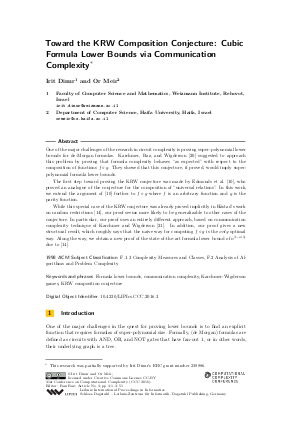Document# Toward the KRW Composition Conjecture: Cubic Formula Lower Bounds via Communication Complexity

### Authors Irit Dinur, Or Meir## File

LIPIcs.CCC.2016.3.pdf
• Filesize: 0.78 MB
• 51 pages

## Cite As

Irit Dinur and Or Meir. Toward the KRW Composition Conjecture: Cubic Formula Lower Bounds via Communication Complexity. In 31st Conference on Computational Complexity (CCC 2016). Leibniz International Proceedings in Informatics (LIPIcs), Volume 50, pp. 3:1-3:51, Schloss Dagstuhl - Leibniz-Zentrum für Informatik (2016)
https://doi.org/10.4230/LIPIcs.CCC.2016.3

## Abstract

One of the major challenges of the research in circuit complexity is proving super-polynomial lower bounds for de-Morgan formulas. Karchmer, Raz, and Wigderson suggested to approach this problem by proving that formula complexity behaves "as expected" with respect to the composition of functions f * g. They showed that this conjecture, if proved, would imply super-polynomial formula lower bounds. The first step toward proving the KRW conjecture was made by Edmonds et al., who proved an analogue of the conjecture for the composition of "universal relations". In this work, we extend the argument of Edmonds et al. further to f * g where f is an arbitrary function and g is the parity function. While this special case of the KRW conjecture was already proved implicitly in Hastad's work on random restrictions, our proof seems more likely to be generalizable to other cases of the conjecture. In particular, our proof uses an entirely different approach, based on communication complexity technique of Karchmer and Wigderson. In addition, our proof gives a new structural result, which roughly says that the naive way for computing f * g is the only optimal way. Along the way, we obtain a new proof of the state-of-the-art formula lower bound of n^{3-o(1)} due to Hastad.
##### Keywords
• Formula lower bounds
• communication complexity
• Karchmer-Wigderson games
• KRW composition conjecture

## Metrics

• Access Statistics
• Total Accesses (updated on a weekly basis)
0